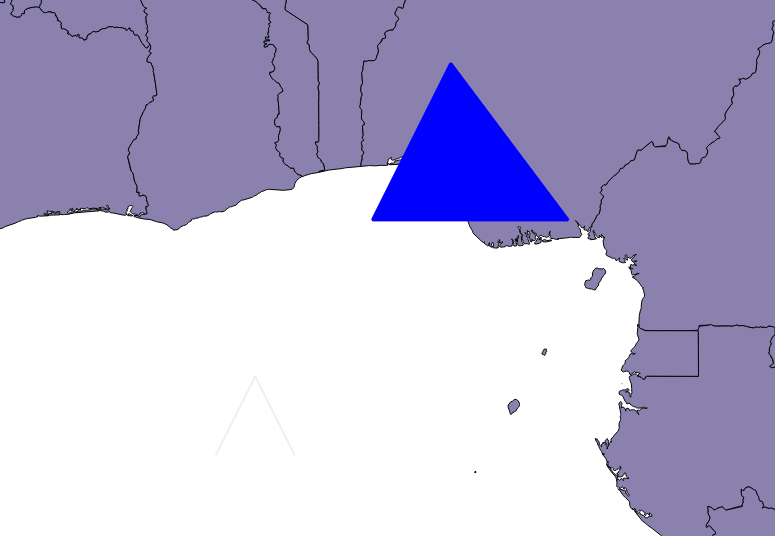qgis-sample-QgsRubberBand.py

``````# coding: utf-8
from PyQt4.QtGui import QColor
from qgis.core import QgsGeometry, QgsPoint
from qgis.gui import QgsRubberBand
from qgis.utils import iface

canvas = iface.mapCanvas()
# To show a polyline
r_polyline = QgsRubberBand(canvas, False)  # False = not a polygon
points = [QgsPoint(-1, -1), QgsPoint(0, 1), QgsPoint(1, -1)]
r_polyline.setToGeometry(QgsGeometry.fromPolyline(points), None)
r_polyline.setWidth(2)

# To show a polygon
r_polygone = QgsRubberBand(canvas, True)  # True = a polygon
points = [[QgsPoint(3, 5), QgsPoint(5, 9), QgsPoint(8, 5)]]
r_polygone.setToGeometry(QgsGeometry.fromPolygon(points), None)

# Customize rubber band
r_polygone.setColor(QColor(0, 0, 255))
r_polygone.setWidth(3)

# Remove both rubber band items
# canvas.scene().removeItem(r_polyline)
# canvas.scene().removeItem(r_polygone)